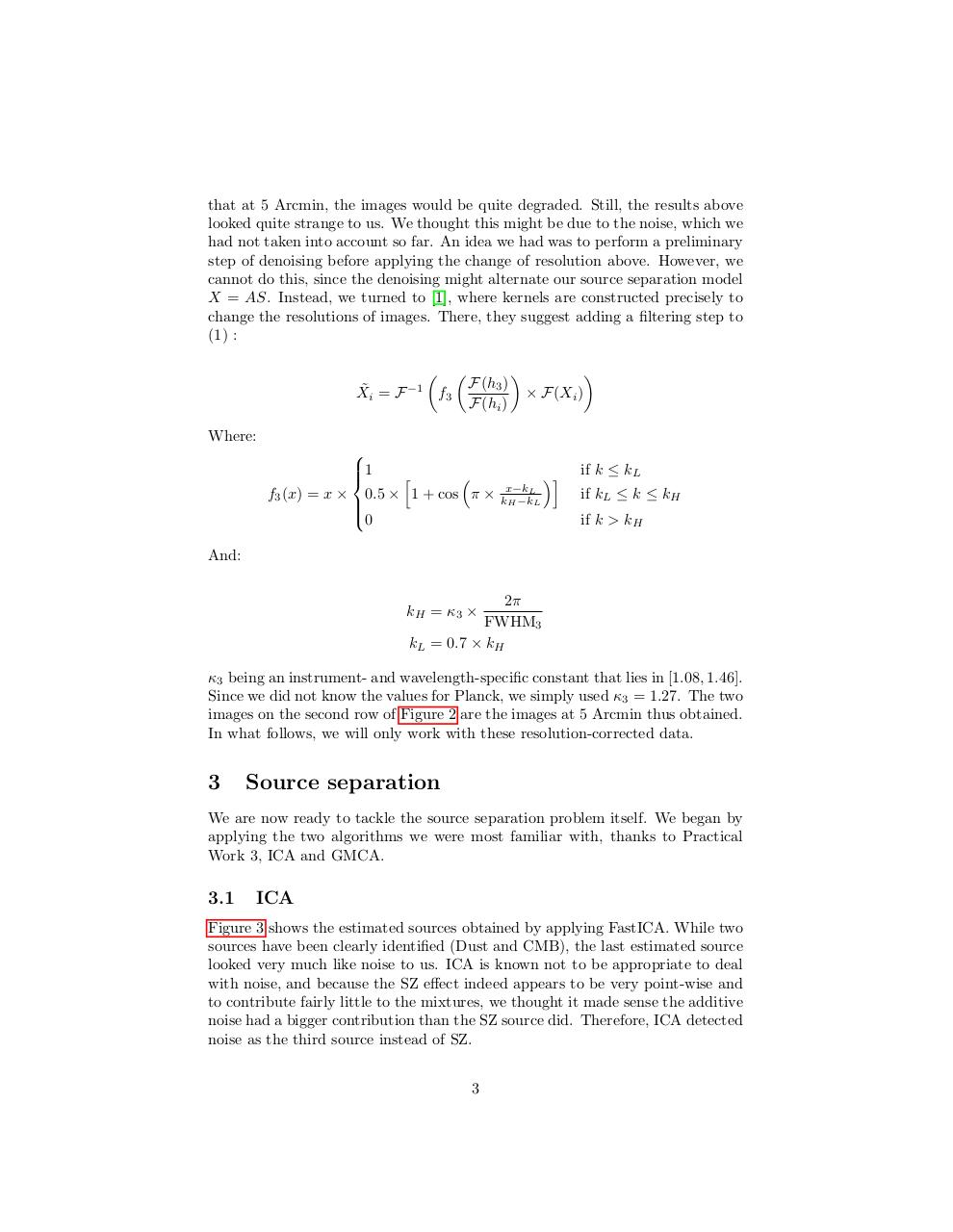# Report SchmitzSitbon.pdfPage 1 2 3 4 5 6 7 8 9 10

#### Text preview

that at 5 Arcmin, the images would be quite degraded. Still, the results above
looked quite strange to us. We thought this might be due to the noise, which we
had not taken into account so far. An idea we had was to perform a preliminary
step of denoising before applying the change of resolution above. However, we
cannot do this, since the denoising might alternate our source separation model
X = AS. Instead, we turned to , where kernels are constructed precisely to
change the resolutions of images. There, they suggest adding a filtering step to
(1) :


 
F(h3 )
× F(Xi )
X˜i = F −1 f3
F(hi )
Where:

1
h

f3 (x) = x × 0.5 × 1 + cos π ×

0

if k ≤ kL
x−kL
kH −kL

i

if kL ≤ k ≤ kH
if k &gt; kH

And:

FWHM3
kL = 0.7 × kH

kH = κ3 ×

κ3 being an instrument- and wavelength-specific constant that lies in [1.08, 1.46].
Since we did not know the values for Planck, we simply used κ3 = 1.27. The two
images on the second row of Figure 2 are the images at 5 Arcmin thus obtained.
In what follows, we will only work with these resolution-corrected data.

3

Source separation

We are now ready to tackle the source separation problem itself. We began by
applying the two algorithms we were most familiar with, thanks to Practical
Work 3, ICA and GMCA.

3.1

ICA

Figure 3 shows the estimated sources obtained by applying FastICA. While two
sources have been clearly identified (Dust and CMB), the last estimated source
looked very much like noise to us. ICA is known not to be appropriate to deal
with noise, and because the SZ effect indeed appears to be very point-wise and
to contribute fairly little to the mixtures, we thought it made sense the additive
noise had a bigger contribution than the SZ source did. Therefore, ICA detected
noise as the third source instead of SZ.
3